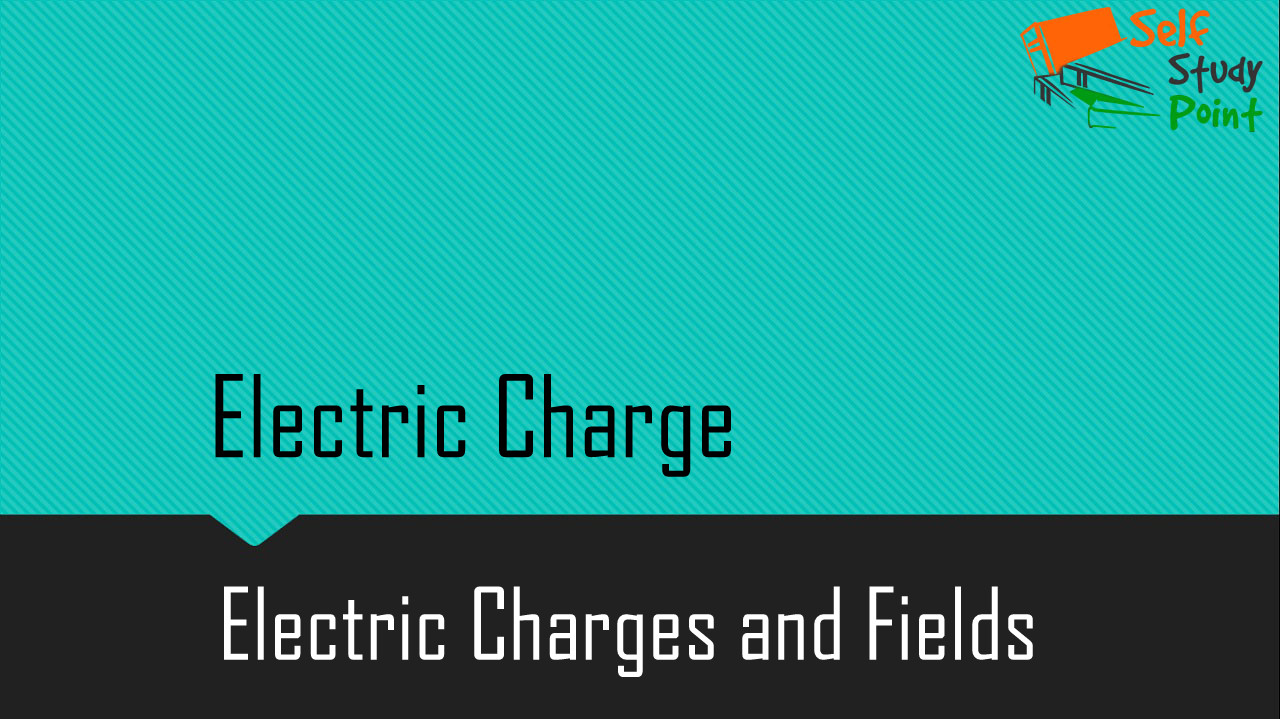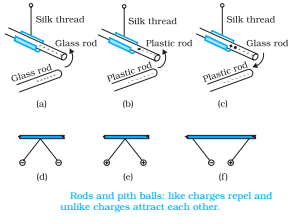Electric charge is the property responsible for electric forces which act between nucleus and electron to bind the atom together. Electric charge is a fundamental property like mass, length etc associated with elementary particles for example electron, proton and much more.

The electric force between two electrons is same as the electric force between two protons kept at the same distance apart i. e., both sets repel each other but electric force between an electron and proton placed at the same distance apart is not repulsive but attractive in nature.## Unit of charge

• The charge on a system can be measured by comparing it with the charge on a standard body.
• SI unit of charge is Coulomb written as C.
• 1 Coulomb is the charge flowing through the wire in 1 second if the electric current in it is 1A.
• The charge on an electron is -1.602 × 10 -19 C and charge on a proton is positive of this value.

## Basic Properties of Electric Charge

Charges add up like real numbers i. e., they are Scalars more clearly if any system has n number of charges q1, q2, q3, qn then total charge of the system is

q = q1 + q2 + q3 + ……………. qn

Proper sign have to used while adding the charges for example if

q1 = +1C
q2 = -2C
q3 = +4C

then the total charge of the system is

q = q1 + q2 + q3
q = (+1) + (-2) + (+4) C
q = (+3) C

### 2. Conservation of charge:

• The charge of an isolated system is conserved.
• The charge cannot be created or destroyed but charged particles can be created or destroyed.

### 3.Quantization of charge

• All free charges are integral multiples of a unit of charge e, where e = -1.602 × 10 -19 C i.e., charge on an electron or proton.
• Thus charge q on a body is always denoted by q = ne where n = any integer positive or negative.

Click on a star to rate it!

Average rating / 5. Vote count:

No votes so far! Be the first to rate this post.